## Collisions

10-22-99

Sections 7.6 - 7.7

### A 2-D collision

Because momentum is a vector, whenever we analyze a collision in two or three dimensions the momentum has to be split up into components. Consider the following example to see how this works. A 1000 kg car traveling at 30 m/s, 30° south of east, collides with a 3000 kg truck heading northeast at 20 m/s. The collision is completely inelastic, so the two vehicles stick together after the collision. How fast, and in what direction, are the car and truck traveling after the collision?

To solve this problem, simply set up two conservation of momentum equations, one for the y-direction (positive y being north) and another for the x-direction (positive x being east). Setting up a vector diagram for the momentum is a good idea, too, like this: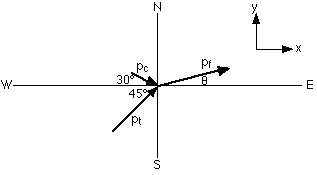To set up the two momentum conservation equations, simply write down the equation for the momentum before the collision in the y-direction and set it equal to the momentum after the collision in the y-direction, and then do the same thing in the x-direction.The y equation can be rearranged to solve for the y component of the final velocity: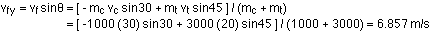Similarly, in the x-direction: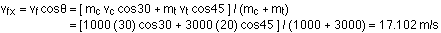It would be easy to figure out the final velocity using the Pythagorean theorem, but let's find the angle first instead, by dividing the y equation by the x equation: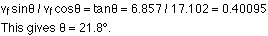Now let's go back to get the final velocity from the Pythagorean theorem :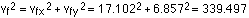This gives a final velocity of 18.4 m/s at an angle of 21.8° north of east.

We could figure out how much energy is lost during the collision if we wanted to; because energy is a scalar rather than a vector, this is done the same way in 2-D (and 3-D) as it is in 1-D.